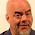## Tuesday, March 31, 2015

### [Pri20150330CPF] How to #Compare #Fractions

Comparing Positive Fractions
How do you compare positive fractions, which are taught in primary (elementary) school?  For example, which is bigger:  5/6  or  3/4 ?

The “orthodox” method is to put them both to a common denominator.  The Lowest Common Multiple (LCM) of  6  and  4  is  12.  Multiplying the left fraction by  2/2  and the right fraction with  3/3  gives, respectively,  10/12  and  9/12.

Since  10/12  >  9/12,  we conclude that  5/6  >  3/4.

Another Method
Here is a “short-cut” that I learned from a schoolmate in primary school.  Basically you “cross-multiply”: multiply the left numerator with the right denominator, and multiply the right numerator with the left denominator, and then compare the products so formed.  That will give you the correct inequality or equality sign (viz. ‘<’, ‘=’ or ‘>’).

As we can see, since  20 > 18,  we conclude that  5/6  >  3/4
Does this method work?  Yes, definitely.  You can try it out with a few pairs of fractions and you can see for yourself that it is so.  Is this method legit?  Why does it work?    I give a formal proof of the method below.

Further Discussion
Use the above method with care.  Some school teachers may not accept the method not because it is not correct, but it sounds “dubious” to them because they have not heard of it or they are not able to prove it for themselves.  Pupils can use this short-cut to give them a quick look-ahead to certain questions, and as a back-up to check their answer after using the Lowest Common Denominator method.  In questions that ask pupils to arrange a few fractions in ascending and descending order, this “crossing method” may give some speed advantage if done carefully.
In primary school, pupils focus on positive fractions.  In secondary school, negative numbers and fractions are introduced.  Does the above trick work for negative fractions?  Was my theorem and proof above carefully phrased enough to cover the negative fractions?
Note that this method works for comparing two fractions at a time only.  Sometimes this cross-multiplying gives rather big numbers.  In that case, it is better to multiply each fractions by the LCM of their denominators.  Essentially this is the same as the orthodox method, except that we do not write the denominators.  Can the above proof be extended to cover this new short-cut?  What do you think?

1.A trick that works in part because test-writers are lazy:

It's extremely common to ask students to compare fractions of the form (m-1)/m and (n-1)/n (like this one, where m=5 and n=4) and for such problems, the greater of m or n goes with the greater of the two fractions, because they are less than 1 by 1/m and 1/n respectively, and the one that is "less by less" must be greater.

It's such a quick formula for producing a question with a known result that I've also seen many testwriters writing comparisons for larger (2 digit, usually greater than 20) denominators use a common integer as the subtrahend, and do the same thing to produce an apparently difficult problem that you can solve instantly if you know the trick: e.g. set common subtrahend to 4, m to 68 and n to 69, and ask students to compare 64/68 and 65/69. (for extra evil, reduce the first fraction, so that the final question is 16/17 versus 65/69, but most testwriters have to produce so many problems in such a short time that they don't hide things that well).

1.Mathematically, the trick works in general. I just used a simple example to illustrate the trick. in Singapore tests questions, pupils don't just get two fractions to compare, they may get a mix of five or six decimals, fractions, mixed numbers and recurring decimals to sort in ascending or descending order.

Note: Only a member of this blog may post a comment.